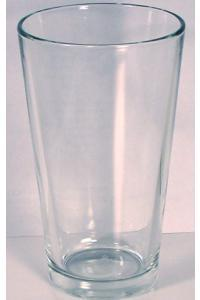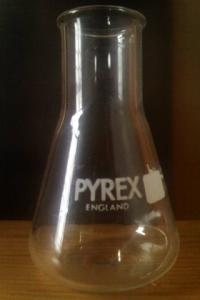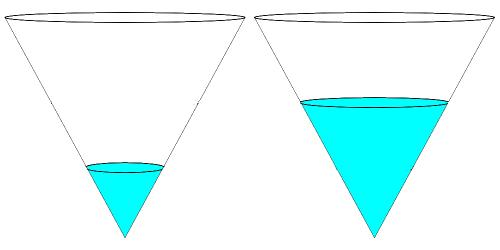#### You may also like### Ball Packing

If a ball is rolled into the corner of a room how far is its centre from the corner?### Three Balls

A circle has centre O and angle POR = angle QOR. Construct tangents at P and Q meeting at T. Draw a circle with diameter OT. Do P and Q lie inside, or on, or outside this circle?### In a Spin

What is the volume of the solid formed by rotating this right angled triangle about the hypotenuse?

# Fill Me up Too

##### Age 14 to 16Challenge Level

In the problem Fill Me Up, we invited you to sketch graphs showing the height of water against the volume for six containers, as they filled up.

Instead of sketching graphs or producing experimental plots, we could produce graphs by analysing the shape of each container and working out the function linking height and volume. Some functions are easier to work out analytically than others!The Pint Glass and the lower portion of the Conical Flask are both frustums of cones.
We can use what we know about the volume properties of a cone to help us to analyse what happens when we fill the pint glass or conical flask with water.

Imagine a cone sitting on its point being filled with water:In the second picture, the height of the water level has doubled. How has the volume of water changed?
What if the height had trebled?
What if the height had increased by a factor of $n$?

How would I need to increase the height in order to double the volume?
How would I need to increase the height in order to treble the volume?
How would I need to increase the height in order to increase the volume by a factor of $n$?

What would a graph of volume (y) against height (x) look like?
What would a graph of height (y) against volume (x) look like?

The Pint Glass is not a whole cone, it is a frustum. How could you use the graph for a cone to work out what the graph for the Pint Glass would look like?

Extension challenge
Using a similar analysis, can you work out the shape of the graph for height against volume for a cone sitting on its base rather than its point?
Can you use your graph to work out what the graph for the Conical Flask would look like?

Very challenging extension:
Can you work out an analytical form for the function linking volume and height for a spherical flask?

Pictures